Engineering Jobs   »   QUIZ : CIVIL ENGINEERING (15-08-2020)

# QUIZ : CIVIL ENGINEERING (15-08-2020)

Quiz: Civil Engineering
Exam: SSC-JE
Topic: MISCELLANEOUS

Each question carries 1 mark
Negative marking: 1/4 mark
Time: 10 Minutes

Q1. The design of eccentrically loaded steel column needs revision if
(a) (f_c^’)/f_c +(f_b^’)/f_b
(b) (f_c^’)/f_c -(f_b^’)/f_b <1
(c) (f_c^’)/f_c +(f_b^’)/f_b <1
(d) (f_c^’)/f_c +(f_b^’)/f_b >1

Q2. Maximum bending moment in roof purlins is taken as
(a) WL/10
(b) WL/20
(c) WL/40
(d) WL/50

Q3. Which of the following loads are to be considered in designing a gantry girder?
(a) (i) and (ii)
(b) (i), (ii) and (iii)
(c) (i) and (iii)
(d) (ii), (iii) and (iv)

Q4. In a plate girder, the shear force in the girder at any section is taken up by:
(a) the web only
(b) the flange only
(c) part of web and part of flange
(d) the whole section

Q5. A vertical plate of a plate girder is called
(a) Web plate
(b) Flange plate
(c) Cover plate
(d) None of these

Q6. In a soil specimen, 60% of soil passing through 4.75 mm IS sieve and 40% of particle are passing through 75 micron IS sieve. Its uniformity coefficient is 5 and coefficient of curvature is 4. As per IS classification, this soil is classified as:
(a) SP
(b) GP
(c) SW
(d) GW

Q7. A point in a compressible flow, where the velocity of fluid is zero, is called:
(a) critical point
(b) vena contracta
(c) stagnation point
(d) none of the above

Q8. A flow in which each liquid particle has a definite path and paths of individual particles do not cross each other, is called a:
(b) uniform flow
(c) stream line flow
(d) non-uniform flow

Q9. A flow through an expanding tube at constant rate is called:

Q10. The ratio of actual discharge of a jet of water to its theoretical discharge is known as
(a) Coefficient of discharge
(b) Coefficient of velocity
(c) Coefficient of contraction
(d) Coefficient of viscosity

SOLUTION

S1. Ans.(c)
Sol. The design of eccentrically loaded steel column needs revision if
▭((f_c^’)/f_c +(f_b^’)/f_b <1)

S2. Ans.(a)
Sol. the maximum bending moment in roof purlins is taken as (‘WL^’)/10 or (‘wL^(2^’ ))/10
W= total load (KN) = wL
S3. Ans.(b)
Sol. the loads which are considered in designing of gantry girders –
S4. Ans.(c)
Sol. in plate girder, the shear force in the girder at any section is taken up by part of web and part of flange. The main purpose of flange plate in plate girders is to resist the bending moment acting on the girder.

S5. Ans.(a)
Sol. A vertical plate of a plate girder is called web plate. The thickness and depth of web plate depends upon bending moment and shear stress acting on the plate.
S6. Ans.(d)
Sol. more than 50% of soil grains are retained over 75 micron sieve then its coarse grained soil.

→ if Cu > 4 than well graded gravel other
S7. Ans. (c)
Sol. In fluid dynamics a stagnation point in a flow field where local velocity of the fluid is zero.

S8. Ans. (c)
Sol.

S9. Ans. (b)
Sol. Flow through an expanding tube is non uniform but rate of flow is constant so steady flow.
S10. Ans. (a)

Sol. coefficient of discharge (c_d) = (Actual velocity )/(theortical velocity )

Sharing is caring!

Thank You, Your details have been submitted we will get back to you.
•How to Prepare for SSC JE 2022 General A...
•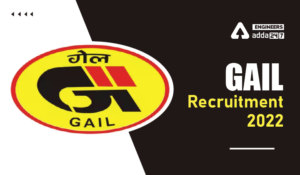GAIL Recruitment 2022, GAIL 282 Non Exec...
•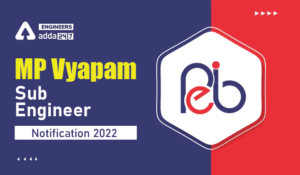MP Vyapam Sub Engineer Notification 2022...
•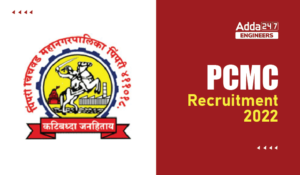PCMC Recruitment 2022, Check here the de...
•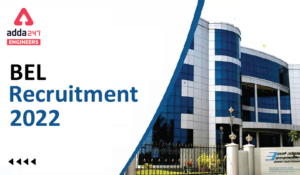BEL Recruitment 2022, Check here For 13 ...
•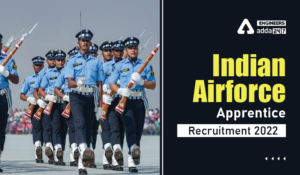Indian Air Force Apprentice Recruitment ...